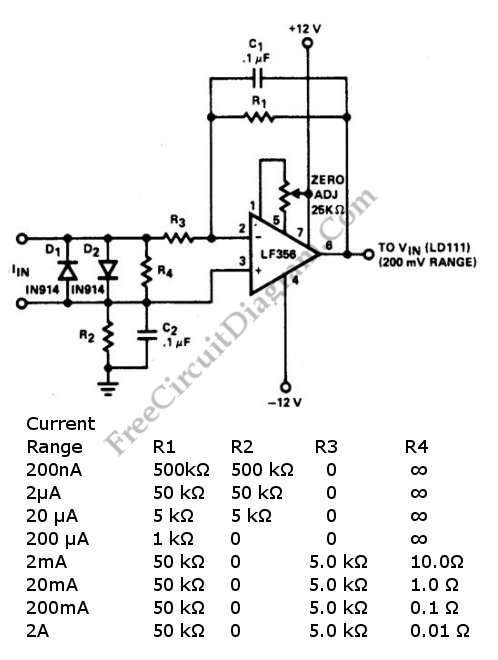# LF356 Wide Range Current-To-Voltage ConverterCurrent to voltage converter circuit can be made using a single resistor, it is very simple since any current will naturally develop a voltage when flow through a resistor. Using an active component,  a small developed voltage on a resistor can be accurately measured since we can amplify it to reach a convenient level to be measured. A schematic diagram below shows a current to voltage converter circuit using op-amp as the active component to amplify the signal. This circuit is suitable to be used for  digital voltmeter (DVM) set to 200.0 mV range.  Using proper resistor value selection, this circuit features eight decades of current range. Here is the schematic diagram of the  circuit:[Circuit’ schematic diagram source: seekic.com]